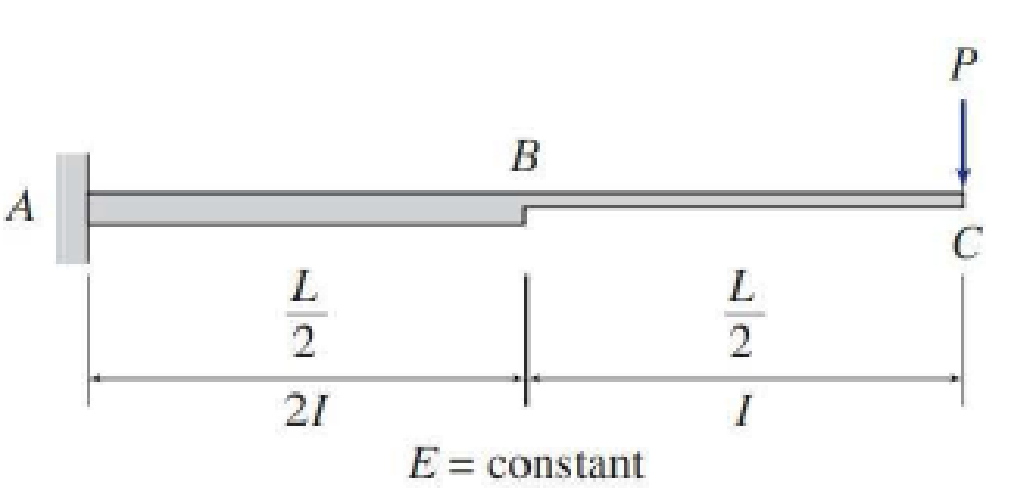# Use the moment-area method to determine the slopes and deflections at points Band C of the beam shown. FIG. P6.14, P6.40

#### Solutions

Chapter
Section
Chapter 6, Problem 14P
Textbook Problem
94 views

## Use the moment-area method to determine the slopes and deflections at points Band C of the beam shown.FIG. P6.14, P6.40

To determine

Find the slope θB&θC and deflection ΔB&ΔC at point B and C of the given beam using the moment-area method.

### Explanation of Solution

Calculation:

Consider elastic modulus E of the beam is constant.

Show the given beam as in Figure (1).

Consider upward is positive and downward is negative.

Consider clockwise is negative and counterclockwise is positive.

Since the point C is free end there is no support reaction at Point C. Therefore, the reaction at point C is also equal to zero.

Determine the reaction at support A using the Equation of equilibrium;

V=0RA=P

Determine the moment at point A using the relation;

MA(P×(L2+L2))=0MA=PL

Determine the moment at point B using the relation;

MB(P×(L2))=0MB=PL2

Show the M/EI diagram for the given beam as in Figure (2).

Elastic curve:

The sign of M/EI diagram is negative, therefore, the beam bends downward. The support A of the given beam is fixed and the slope at A is zero. Therefore, the tangent to the elastic curve at A is horizontal.

Show the elastic curve diagram as in Figure (3).

The slope at point B can be calculated by evaluating the change in slope between A and B.

Express the change in slope using the first moment-area theorem as follows:

θB=θBA=AreaoftheM/EIbetweenAandB=Areaoftriangle+Areaofrectangle=12×b×h+(b×h)

Here, b is the width and h is the height of the respective triangle and rectangle.

Substitute L2 for b and PL4EI for h.

θB=θBA=12×L2×(PL4EI)+(L2)×(PL4EI)=PL216EI+PL28EI=PL2+2PL216EI=3PL216EI

Hence, the slope at point B is 3PL216EI(Clockwise)_.

The deflection of B with respect to the undeforemd axis of the beam is equal to the tangential deviation of B from the tangent at A.

Express the deflection at B using the second moment-area theorem as follows:

ΔB=ΔBA=2PL3+3PL396EI=5PL396EI

Hence, the deflection at B is 5PL396EI()_

### Still sussing out bartleby?

Check out a sample textbook solution.

See a sample solution

#### The Solution to Your Study Problems

Bartleby provides explanations to thousands of textbook problems written by our experts, many with advanced degrees!

Get Started

Find more solutions based on key concepts
Given matrices: [A]= [B]=, and {C}={124}, perform the following operations. a. [A]+[B]=? ...

Engineering Fundamentals: An Introduction to Engineering (MindTap Course List)

Are top managers likely to be more effective if they have IT experience? Why or why not?

Systems Analysis and Design (Shelly Cashman Series) (MindTap Course List)

Explain the need for the two-phase commit protocol. Then describe the two phases.

Database Systems: Design, Implementation, & Management

What is the purpose of the master cylinder vent port?

Automotive Technology: A Systems Approach (MindTap Course List)

List three types of screwdriver tips.

Precision Machining Technology (MindTap Course List)

cookie a. compromised computer or device whose owner is unaware the computer or device is being controlled remo...

Enhanced Discovering Computers 2017 (Shelly Cashman Series) (MindTap Course List)

What does the AWS abbreviation “SENSE” stand for?

Welding: Principles and Applications (MindTap Course List)

If your motherboard supports ECC DDR3 memory, can you substitute non-ECC DDR3 memory?

A+ Guide to Hardware (Standalone Book) (MindTap Course List)